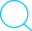2018-04-02

# 精密空调制冷量的计算## 制冷量简便计算方法

### 方法一：功率及面积法

$Q_t = Q_1 + Q_2$
• Qt 总制冷量(kw)
• Q1 室内设备负荷 ( $= 设备功率 \times 0.8$ )
• Q2 环境热负荷 ( $=0.18(KW/m^2) \times 机房面积$ )

### 方法二：面积法 (当只知道面积时)

$Q_t = S \times p$
• Qt 总制冷量 (kw)
• S 机房面积 (m2)
• P 冷量估算指标

#### 精密空调场所冷负荷估算指标

• 电信交换机、移动基站 (350-450W/m2)
• 金融机房 (500-600W/m2)
• 数据中心 (600-800W/m2)
• 计算机房、计费中心、控制中心、培训中心 (350-450W/m2)
• 电子产品及仪表车间、精密加工车间 (300-350W/m2)
• 保准检测室、校准中心 (250-300W/m2)
• Ups 和电池室、动力机房 (300-500W/m2)
• 医院和检测室、生活培养室、洁净室、实验室 (200-250W/m2)
• 仓储室（博物馆、图书馆、档案馆、烟草、食品） (150-200W/m2)

#### UPS机房空调选项计算

1. $BTU/小时 = KCal \times 3.96$
2. $KCal = KVA \times 860$
3. $BUT/小时 = KVA(UPS容量) \times 860 \times 3.96 \times (1-UPS效率) = KVA(UPS容量) \times 3400(1-UPS效率)$

$10KVA \times 3400 \times (1-0.85) = 5100 BTU/小时$

1英热单位/时(BTU/h) = 0.293071瓦(W)

#### IDC机房空调选项计算公式

$Q = W \times 0.8 \times (0.7 \text{~} 0.95) + \frac {(80 \text{~} 200) \times S}{1000}$
• Q为制冷量，单位KW；
• W为设备功耗，单位KW；按用户需求暂按110KW；
• 0.8为功率因数；
• 0.7~0.95为发热系数，即有多少电能转化为热能；取0.7；
• 80~200是每平方米的环境发热量，单位是W；
• S为机房面积，单位是m2

## 根据不同情况确定制冷量

### 情况二（对机房设备等情况考察之下）#### 1：机房主要热量的来源

1. 设备负荷（计算机及机柜热负荷）；
2. 机房照明负荷；
3. 建筑维护结构负荷；
4. 补充的新风负荷；
5. 人员的散热负荷等。
6. 其他

#### 2：热负荷分析

（1）计算机设备热负荷

$Q_1 = 860 \times P \times η1η2η3 \quad \text {Kcal/h}$
• Q1：计算机设备热负荷
• P：机房内各种设备总功耗
• η1：同时使用系数
• η2：利用系数
• η3 ：负荷工作均匀系数通常

η1η2η3取0.6—0.8之间，本设计考虑容量变化要求较小，取值为0.6。

（2）照明设备热负荷

$Q_2 = C \times P \quad \text {Kcal/h}$
• P：照明设备标定输出功率
• C：每输出1W放热量Kcal/hw（白炽灯0.86，日光灯1）

（3）人体热负荷

$Q_3 = P \times N \quad \text {Kcal/h}$
• N：机房常有人员数量
• P：人体发热量，轻体力工作人员热负荷显热与潜热之和，在室温为21℃和24℃时均为102Kcal。

（4）围护结构传导热

$Q_4 = K \times F \times (t_1-t_2) \quad \text {Kcal/h}$
• K：转护结构导热系统，普通混凝土为1.4~1.5
• F：转护结构面积
• t1：机房内内温度℃
• t2：机房外的计算温度℃

（5）新风热负荷计算较为复杂，在此方案中，我们以空调本身的设备余量来平衡，不另外计算。

（6）其他热负荷除上述热负荷外，在工作中使用的示波器、电烙铁、吸尘器等也将成为热负荷，由于这些设备功耗小，只粗略根据其输入功率与热功当量之积计算。 $Q_5=860xP$